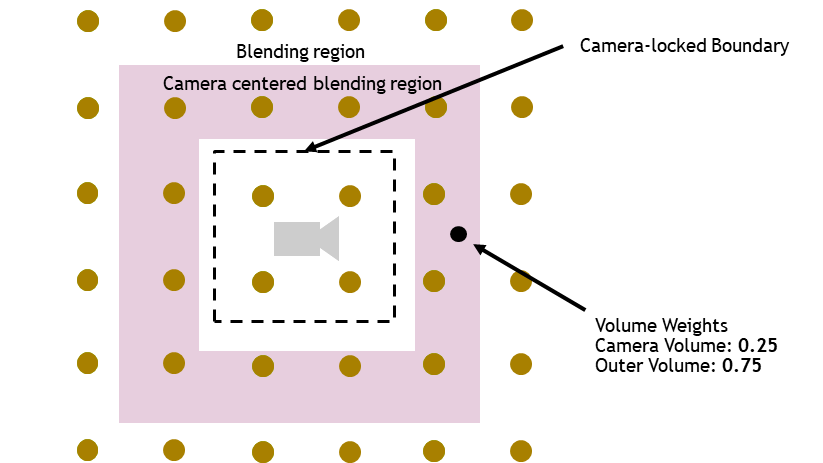# Precomputed Probe## 预放置 probes

### 四面体镶嵌（Tetrahedral Tessellations）

ps：将这些 probe 进行三角化（将空间切分成四面体）需要用到 Delaunay Triangulation 完美三角剖分算法。• 手动放置的方式比较费人力
• 运行时，查找 shading point 所处四面体的运算量较高：四面体的分布并不是均匀的，最糟糕没有优化的情况下需要暴力遍历每一个四面体判断 shading point 是否在该四面体里面，无论对 CPU 还是 GPU 运算都不太理想### Indirect Light Cache

Indirect Light Cache, 即 ILC（间接光缓存），是 UE4 的方案，它基本上是在静态物体表面法线向上自动地均匀放置 probe ，这是基于假设受光照影响最大的地方都是在靠近静态物体的地方。

• ILC 通过 CPU 寻找物体周围的 probe：所有的 probe 的数据将保存到八叉树中以方便物体找到周围的 probe### Volumetric Light Map

Volumetric Light Map, 即 VLM（体积光照贴图），也是 UE4 的方案，它使用网格（Grid）采样点保存 probe 数据，而在静态物体表面附近，会对网格进一步细分。

• VLM 通过 GPU 来寻找物体周围的 probe ：所有 probe 的数据将烘焙至贴图中，不同细分层度的网格将使用 level 不同的贴图，这样可以方便地在 GPU 中进行逐像素的三线性插值

• VLM 比 ILC 的 probe 要多更多，从而 VLM 的 GI 效果更佳，但要存储的数据更多

• VLM 的存储结构决定了可以通过 GPU 算法来寻找 probe，这比传统的 CPU 算法性能更加可观（即便 VLM probe 数量要多得多）

• VLM 更适合将 probe 应用到体积雾效果中，这是因为 ILC 往往不在几乎没什么物体的空间放置 probeUE 4.18 以后使用 VLM 代替了 ILC 方法作为 UE4 默认的 probe 方案。

ILC vs. VLM 效果图：## 烘焙光照信息

### SH lighting1. 根据 shading point 的世界坐标找到其邻近的 probes
2. 对这些 probes 的 SH 系数以距离为权重进行混合，得到 shading point 的 SH 系数（比较粗糙的光照信息）
3. 根据 SH 系数就可以重建 shading point 的光照信息信息

## 优缺点

• 预计算能够节省大量的计算来实现还可以的 GI 效果

• 由于是预计算，因此不支持动态光照
• 对于大场景需要很多的存储空间，并需要设计一定复杂程度的空间结构来管理查询

# DDGI（or RTXGI）

Dynamic Diffuse Global Illumination（DDGI），则提供了一种支持动态光照的 probe 方案。

NVIDIA 的 DDGI 实现版本则一般称之为 RTXGI 技术，因此基本上说起 RTXGI 就是相当于在说 DDGI

## 动态/预放置 probes

### DDGI volume

DDGI volume：一个立方体积区域，里面均匀放置着 probes（类似于 grid），在 DDGI Volume 内的物体就能被间接光照亮，实现 GI 效果。虽然 volume 也需要手动放置，但是粒度更大，比起手动放置 probe 工作量要少得多。

• volume 既可以预先固定在某个位置（静态放置），也可以动态改变位置（动态放置）
• 动态放置 volume：需要实时探测几何信息 + 光照信息
• 静态放置 volume：需要实时探测光照信息，而对于几何信息则可以选择预计算或者实时探测

• 对于静态放置：会先放置一个大号的静态 volume 囊括整个场景（常用于表现室外间接光），然后再在场景中各个房间都放置一个静态 volume（更加高清晰度表现室内间接光）

• 对于动态放置：往往会多个大小不一的 volumes 绑定到 camera 上，这样就可以跟随 camera 移动对视野内的一定范围的物体应用间接光照，并且具有质量上的 LOD 效果（近处采用高精度 volume，远处采用低精度 volume）

• 在嵌套多层的 volume 之间会做一个线性淡出从而避免明显的边界## 探测动态光照信息

DDGI probe 采取 ray tracing 的方式去实时探测动态光照信息，并且使用了 八面体映射（octahedral mapping） 技术来缓存收集到的光照信息：将球面方向映射成一张 2D texture，一个 texel 对应一个立体角方向，texel 的值即代表了其对应立体角方向的值。RTXGI 实现中，ray tracing 就需要使用到硬件光追 API（需要 RTX 系列显卡）

DDGI probe 的数据组成：

• 6×6 irradiance texture：存储每个 texel 方向的 irradiance $$E(\omega)$$
• 14×14 moment texture：存储每个 texel 方向的深度 $$r(w)$$ 及深度的平方 $$r^2(w)$$，以便进行 variance depth test

variance depth test 在下文 light leaking 问题中再详细介绍。

1. probe 均匀向球面上射出若干个 rays，并记录每个 hit point 的 position，normal，albedo 并将结果存入分别存入三张 texture

1. one-bounce lighting：通过 position，normal，albedo, rayDir 进行 hit point 的直接光照着色

$L_{one} = \sum_{lights} (L_{light} * f * \cos \theta)$

1. multi-bounce lighting：通过 position，normal 并利用周围 probes 的上帧 irradiance texture 来重建 hit point 的光照信息，并进行间接光照着色

$L_{multi} = \frac{E(position, normal)* albedo}{\pi}$

$L(rayDir) = L_{one} + L_{multi}$

$E(texelDir) = \frac{\sum_{\text {rays}} L(rayDir)\cdot \max (0, \cos \theta )}{\sum_{\text {rays}}\max (0, \cos \theta )}$

1. 使用 position texture 来更新 probe 中的 moment texture

\begin{aligned} r(texelDir) &=\frac{\sum d(rayDir) \cos ^s \theta_i}{\sum \cos ^s \theta_i} \\ r^2(texelDir) &=\frac{\sum d(rayDir)^2 \cos ^s \theta_i}{\sum \cos ^s \theta_i} \end{aligned}

position texture 代表了某个方向的光线交点的世界空间坐标位置，通过变换可以计算成该方向的最近 depth，这将在后面用于 probe 的 depth test，去解决一些漏光问题。

1. temporal filtering：

$E_{new}(texelDir)=\operatorname{lerp}(E_{old}(texelDir),E(texelDir), historyWeight )$1. 根据 shading point 的世界坐标找到其邻近的 8 个 probes

2. 对每个相邻的 probe：

• 计算出 shading point 到 probe 的 $$dir$$

• 测试 $$dir$$ 是否与 $$n$$ 面向同一侧：若 $$dot(dir, n)\le 0$$，则跳过该 probe

• 根据 $$dir$$ 索引到 texture 中对应的 texel ，得到 $$E(dir)$$ , $$r(dir)$$$$r^2(dir)$$

• 计算 shadow 系数：根据 $$r(dir)$$$$r^2(dir)$$ 来对 shading point 进行类似 shadow map 的测试

• 该 shading point 受到的光照信息 irradiance 即为 $$E(dir)*shadow$$

## 优缺点

• 支持动态光照信息
• 在预计算模式下，还能避免运行时 ray tracing 的开销
• 若将 volume 绑定在 camera 上，则存储空间的开销增长与场景大小无关

• 硬件 ray tracing 的开销可能仍然很大
• 在预计算模式下，对于大量动态物体的场景可能会很容易穿帮，因为几何信息只包含静态物体
• 不能放置太多 probe，因为每个 probe 需要更多存储空间了（textures 相比于 SH 系数）# Screen Space Probe

## 动态放置 probes

### uniform placement

Uniform：在屏幕上均匀放置 probe；一般使用 2d texture 存放 uniform probes。UE5 Lumen 就是采取了 screen space probe 的方案。

## 探测动态光照信息## 优缺点

• 对性能友好：基于屏幕的方案所需要的 probes 数量明显要少很多
• probe 的利用率更高：贴合在物体表面上的 probes 离物体表面的距离更近，因此上 irradiance 的表示更加贴切和准确；而在空间中均匀放置的 probes 可能会有一些压根没怎么用到，利用率更低

• 能量损失更严重：ray 命中的地方很可能不存在 screen space probe，即丢失了屏幕可见几何表面以外的光照信息。因此，一般来说 screen space probe 会搭配别的 GI 方法补上这块的空缺。

# Light Leaking 问题## 标记法$d_x = x_{probe} - x \\dir = normalize(x-probe)$

$$x_{probe}$$ 为 probe 的位置，$$x$$ 为 shading point 的位置。

$shadow = d_x > d(dir)?0:1$

$\mu = r(dir)\\ \sigma = r^2({dir})-r(dir)*r(dir)$

$shadow = P(X>d_x) \approx \frac{\sigma^{2}}{\sigma^{2}+(d_x-\mu)^{2}}$

# 参考

posted @ 2022-10-26 14:35  KillerAery  阅读(645)  评论(0编辑  收藏  举报